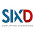Programming, tutorials, mechatronics, operating systems, and other tech stuff

# How to make ip address calculator with java netbeans

This one is a video tutorial. And here is the source code which is used in the tutorial.
```For step-by-step instruction, please watch the video. ```
``````int prefix = Integer.valueOf(mask.getText());
int pos = prefix / 8; //important octet position
int rem = prefix % 8; //binary digit of importan octet

String binIpFull = "";
String subBinary = "";
String subBinaryFull = "";
String binnetidFull = "";
String binbcidFull = "";
String netidFull = "";
String bcidFull = "";

//loop to generate binary mask from octet pos
for (int i = 1; i & lt; = 8; i++) {
if (i & lt; = rem) {
subBinary += "1";
} else {
subBinary += "0";
}
}

//ip octets array
int[] oct = {
Integer.valueOf(oct1.getText()),
Integer.valueOf(oct2.getText()),
Integer.valueOf(oct3.getText()),
Integer.valueOf(oct4.getText())
};

//binary of important octet
String binOct = String.format("%8s", Integer.toBinaryString(oct[pos])).replace(' ', '0');

String binNetId = "";
String binBcId = "";

for (int i = 0; i & lt; 8; i++) {
String binPointer = subBinary.substring(i, i + 1);
if (binPointer.equals("1")) {
binNetId += binOct.substring(i, i + 1);
binBcId += binOct.substring(i, i + 1);
} else {
binNetId += "0";
binBcId += "1";
}
}

for (int i = 0; i & lt; = 3; i++) {
binIpFull += String.format("%8s", Integer.toBinaryString(oct[i])).replace(' ', '0');
if (i < pos) { = ""
subbinaryfull += "11111111"; = ""
binnetidfull = ""
','
0 ');="" binbcidfull="" netidfull="" bcidfull="" ="" }else="" if(i="">pos){
subBinaryFull += "00000000";
binnetidFull += "00000000";
binbcidFull += "00000000";
netidFull += "0";
bcidFull += "1";
} else {
subBinaryFull += subBinary;
binnetidFull += binNetId;
binbcidFull += binBcId;
netidFull += Integer.parseInt(String.valueOf(binNetId), 2);
bcidFull += Integer.parseInt(String.valueOf(binBcId), 2);
}
if (i != 3) {
subBinaryFull += ".";
binnetidFull += ".";
binbcidFull += ".";
netidFull += ".";
bcidFull += ".";
binIpFull += ".";
}
}
subBinLabel.setText(subBinaryFull);
netBinLabel.setText(binnetidFull);
bcBinLabel.setText(binbcidFull);
netIdLabel.setText(netidFull);
bcIdLabel.setText(bcidFull);
ipBinLabel.setText(binIpFull);
``````
``` ```

You can watch the video here:

1.Great job.... Awesome list, just starting a blog and this is going to be a massive help. Thank you!

Node JS Training in Chennai
Node JS Training

1.2.Nice post! This is a very nice blog that I will definitively come back to more times this year! Thanks for informative post. https://192-168-i-i.com

3.mortgage calculator

4.This comment has been removed by the author.

5.IP delivers are doled out to a host either over again after booting time or for all time through fixed design of its product or equipment. Utilizing a "static Internet Protocol address" signifies diligent design. Utilizing a "unique Internet Protocol address," then again, is doling out a PC's location from time to time. 192.168.l0.1

6.7.8.This is most informative and also this post most user friendly and super navigation to all posts.
Dimensional Control
AS Built Drawings in India

9.Grab the best AWS Training in Chennai from Infycle Technologies, the best software training institute, and Placement centre in Chennai. We also provide technical courses like Power BI, Cyber Security, Graphic Design and Animation, Block Security, Java, Oracle, Python etc. For free demo class and enquiry call 7504633633.